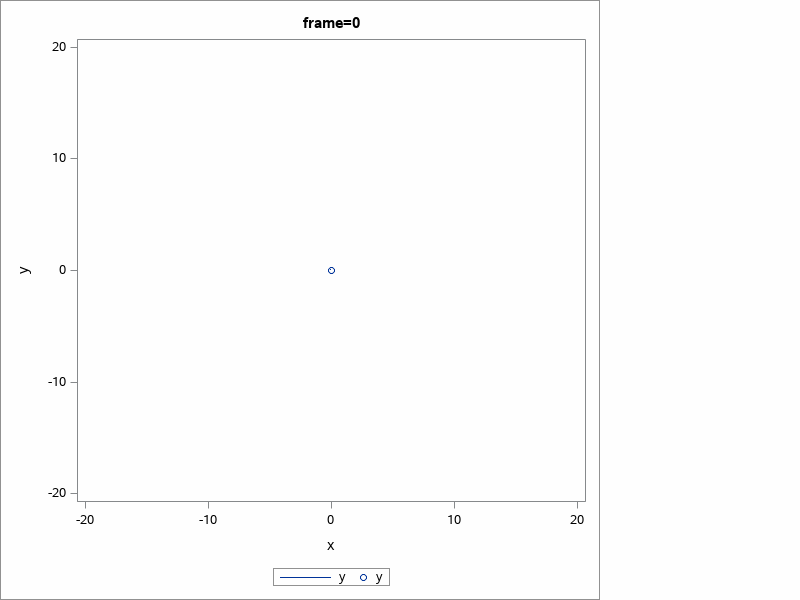## Any options to create a compressed or smaller animated GIF ?

Consider this program that creates an animated gif.  The file size is ~1.25MB

It can be compressed about 83% by external processes (https://gifcompressor.com/)

Are there any SAS options for directly creating a compressed anim gif ?

Program

Spoiler
```data have;
do angle = 0 to 1080 by 15;
theta = 2 * constant('PI') / 360 * angle;
r = theta;
x = r * cos(theta);
y = r * sin(theta);
output;
end;
run;

filename animgif "spiral.gif";
filename htmlout "spiral.html";   /* HTML output */

proc stream outfile=htmlout; BEGIN
<IMG src='spiral.gif'>
</BODY></HTML>
;;;;

ods _all_ close;

ods graphics / width=600 height=600;
*ods graphics / imagename="spiral" reset=index(1) outputfmt=gif;

options
printerpath=gif
animation=start
animduration=0.10
animloop=yes
noanimoverlay
;

ods printer file=animgif;
options animation=start;

proc sql;
create table spiral as
select each.angle as frame, self.x, self.y, self.theta
from have as self join have as each
on each.theta >= self.theta
order by frame, self.theta
;

proc sgplot data=spiral;
by frame;
series x=x y=y;
scatter x=x y=y / markerattrs=(symbol=circle);
xaxis min=-20 max=20;
yaxis min=-20 max=20;
run;

proc sort data=spiral;
by descending frame theta;
run;

proc sgplot data=spiral;
by descending frame;
series x=x y=y;
scatter x=x y=y / markerattrs=(symbol=circle);
xaxis min=-20 max=20;
yaxis min=-20 max=20;
run;

options animation=stop;
ods printer close;```3 REPLIES 3

## Re: Any options to create a compressed or smaller animated GIF ?

You haven't specified a resolution/DPI. If you do, does that change the file size?

## Re: Any options to create a compressed or smaller animated GIF ?

Turns out GIF device is not so easy.  The GIF dpi is 96.

Setting the Resolution of Your Graph doc says:

Note: Devices other than those listed (PNG, PNGT, PNG300, SVG, SVGT, SVGVIEW, or SVGZ) do not honor the IMAGE_DPI= option.

Using the XPIXELS=, XMAX=, YPIXELS=, and YMAX= Graphics Options to Set the Resolution for the Traditional Devices
The resolution of GIF and BMP images is fixed and cannot be changed using this method.

The only improvement I could get was to ensure the papersize matched the ods graphics size.

`ods graphics  / width=4in height=4in ;`

``` options papersize = ("4in",     "4in")```

`        printerpath=gif`

`        animation=start`

`        animduration=0.10`

`        animloop=yes`

```        noanimoverlay ;```Ksharp
Super User

## Re: Any options to create a compressed or smaller animated GIF ?

Yeah. as Reeza said, Try option dpi=200 .
Discussion stats
• 3 replies
• 401 views
• 0 likes
• 3 in conversation